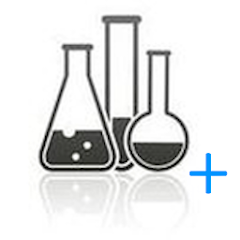# AlcoholCalculator+

500+Everyone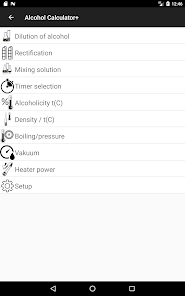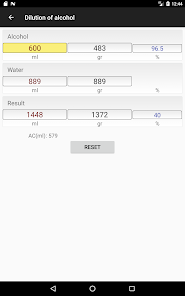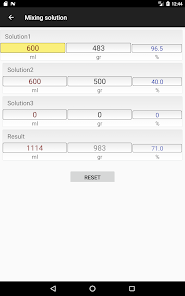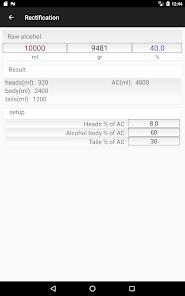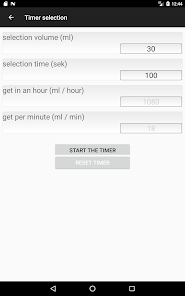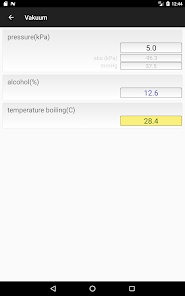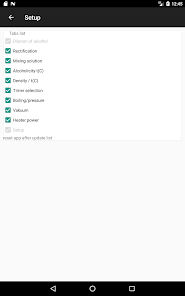Calculator for alcohol dilution (calculation of the required amount of alcohol and water to obtain an alcohol mixture of the desired strength).
The calculation is made taking into account the contraction (change in the total volume when mixing two liquids).
+ calculation of mixing of two/three solutions of varying degree of alcohol content.
+ calculation of dilution by weight.
+ calculation of the alcohol content of the solution in the cube and the steam temperature in the cube.
+ calculation of density change from temperature.
+ calculation of alcohol content from the boiling point, taking into account ATM.pressures.
+ calculation of mixing cold and hot water to obtain the desired temperature and volume.
+ timer calculation selection for a given time (for example, the selection of goals in ml / hour)
+ tab for calculating the dissolution of sugar in water.
+Added the heating POWER CALCULATION tab

Updated on
Feb 3, 2021

## Data safety

Safety starts with understanding how developers collect and share your data. Data privacy and security practices may vary based on your use, region, and age. The developer provided this information and may update it over time.No data shared with third parties